EL10C TUTORIAL #2   Saturday, 28 June 2003

1. Calculate ID(Q) and VGS(Q)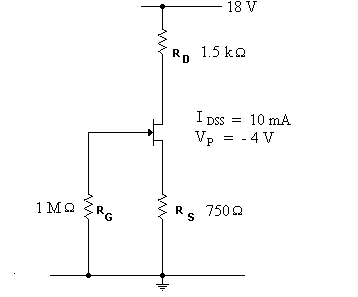2. Calculate ID(Q) and VGS(Q)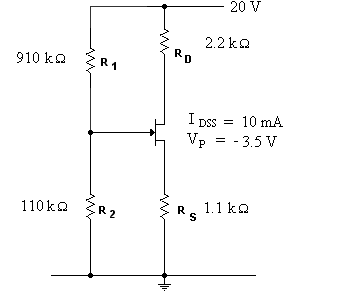3. Draw the equivalent circuit and hence determine the voltage gain (please note... gfs = gm .... because gm is just another way of writing the TRANSCONDUCTANCE... which I gave you as gfs in class.... the formula for finding transconductance is HERE)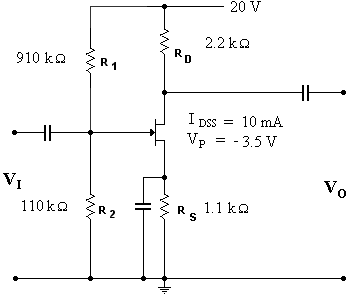4. Try to draw the equivalent circuit for the bootstrap circuit below.... and if you can, calculate the voltage gain.... hie is the input base-emitter resistance and hoe is the collector-emitter output conductance.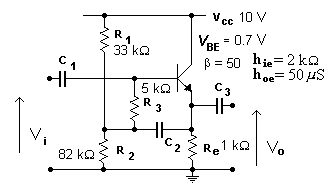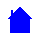EL10C Homepage